# Siteswap facts for kids

Kids Encyclopedia Facts

Siteswap, also called quantum juggling or the Cambridge notation, is a numeric juggling notation used to describe or represent juggling patterns. The term may also be used to describe siteswap patterns, possible patterns transcribed using siteswap. Throws are represented by positive integers that specify the number of beats in the future when the object is thrown again: "The idea behind siteswap is to keep track of the order that balls are thrown and caught, and only that." It is an invaluable tool in determining which combinations of throws yield valid juggling patterns for a given number of objects, and has led to previously unknown patterns (such as 441). However, it does not describe body movements such as behind-the-back and under-the-leg. Siteswap assumes that "throws happen on beats that are equally spaced in time."

For example, a three-ball cascade may be notated "3 ", while a shower may be notated "5 1".

## Origin

The notation was invented by Paul Klimek in Santa Cruz, California in 1981, and later developed by undergraduates Bruce "Boppo" Tiemann and the late Bengt Magnusson at the California Institute of Technology in 1985, and by Mike Day, mathematician Colin Wright, and mathematician Adam Chalcraft in Cambridge, England in 1985 (whence comes an alternative name). The numbers derive from the number of balls used in the most common juggling patterns. Siteswap has been described as, "perhaps the most popular" name.

The name siteswap comes from the ability to generate patterns by "swapping" landing times of any 2 "sites" in a siteswap using the swap property. For example, swapping the landing times of throws "5" and "1" in the siteswap "51" generates the siteswap "24".

## Vanilla

Its simplest form, sometimes called vanilla siteswap, describes only patterns whose throws alternate hands and in which one ball is thrown from each hand at a time. If one were juggling while walking forward, something like the adjacent diagram would be seen from above, sometimes called a space-time diagram or ladder diagram. In this diagram, three balls are being juggled. Time progresses from the top to the bottom.

This pattern can be describe by stating how many throws later each ball is caught. For instance, on the first throw in the diagram, the purple ball is thrown in the air (up out of the screen, towards the bottom left) by the right hand, next the blue ball, the green ball, the green ball again, and the blue ball again and then finally the purple ball is caught and thrown by the left hand on the fifth throw, this gives the first throw a count of 5. This produces a sequence of numbers which denote the height of each throw to be made. Since hands alternate, odd-numbered throws send the ball to the other hand, while even-numbered throws send the ball to the same hand. A 3 represents a throw to the opposite hand at the height of the basic three-cascade; a 4 represents a throw to the same hand at the height of the four-fountain, and so on.

Siteswap Throw Names
Throw Name Beats object is in Air Switches hands Description
0 - - Empty hand
1 1 Yes Throw from one hand to the other
2 0 No Momentary hold
3 3 Yes Throw from a 3 ball cascade
4 4 No Throw from a 4 ball fountain
5 5 Yes Throw from a 5 ball cascade
6 6 No Throw from a 6 ball fountain
7 7 Yes Throw from a 7 ball cascade
8 8 No Throw from a 8 ball fountain
9 9 Yes Throw from a 9 ball cascade
a 10 No Throw from a 10 ball fountain
b 11 Yes Throw from a 11 ball cascade
... ... ... ...

There are three special throws: a 0 is a pause with an empty hand, a 1 is a quick pass straight across to the other hand, and a 2 is a momentary hold of an object. Throws longer than 9 beats are given letters starting with a. The number of beats a ball is in the air usually corresponds to how high it was thrown, so many people refer to the numbers as heights, but this is not technically correct; all that matters is the number of beats in the air, not how high it is thrown. For example, bouncing a ball takes longer than a throw in the air to the same height, and so can be a higher siteswap value while being a lower throw.

Each pattern repeats after a certain number of throws, called the period of the pattern. The period is the number of digits in the shortest non-repeating representation of a pattern. For example, the pattern diagrammed on the right is 53145305520 which has 11 digits and therefore has a period of 11. If the period is an odd number, like this one, then each time the sequence is repeated, the sequence starts with the other hand, and the pattern is symmetrical because each hand is doing the same thing (although at different times). If the period is an even number then on every repeat of the pattern, each hand does the same thing it did last time and the pattern is asymmetrical.

The number of balls used for the pattern is the average of the throw numbers in the pattern. For example, 441 is a three-object pattern because (4+4+1)/3 is 3, and 86 is a seven-object pattern. All patterns must therefore have a siteswap sequence that averages to an integer. Not all such sequences describe patterns; for example 543 with integer average 4 but its three throws all land at the same time, colliding.

Some hold to a convention in that a siteswap is written with its highest numbers first. One drawback to doing so is evident in the pattern 51414, a 3-ball pattern which cannot be inserted into the middle of a string of 3-throws, unlike its rotation 45141 which can.

## Synchronous

Siteswap notation can be extended to denote patterns containing synchronous throws from both hands. The numbers for the two throws are combined in parentheses and separated by a comma. Since synchronous throws are only thrown on even beats, only even numbers are allowed. Throws that move to the other hand are marked by an x following the number. Thus a synchronous three-prop shower is denoted (4x,2x), meaning one hand continually throws a low throw or 'zip' to the opposite hand, while the other continually makes a higher throw to the first. Sequences of bracketed pairs are written without delimiting markers. Patterns that repeat in mirror image on the opposite side can be abbreviated with a *. For example, Instead of (4,2x)(2x,4) (3-ball box pattern), can be abbreviated to (4,2x)*.

## Multiplexing

A further extension allows siteswap to notate patterns involving multiple throws from either or both hands at the same time in a multiplex pattern. The numbers for multiple throws from a single hand are written together inside square brackets. For example, 33 is a normal 3-ball cascade, with a pair of balls always thrown together.

## Passing

Simultaneous juggling: <xxx|yyy> notation means one juggler does 'xxx' while another does 'yyy'. 'p' is used to represent a passing throw. For example, <3p 3|3p 3> is a 6 prop '2 count' passing pattern, where all left hand throws are passes and right hand throws are selves. This can also be used with synchronous patterns; a two-person 'shower' is then <(4xp,2x)|(4xp,2x)>

If the pattern contains fractions, e.g. <4.5 3 3 | 3 4 3.5> the juggler after the bar is supposed to be half a count later, and all fractions are passes. If both juggle the same pattern (although shifted in time), the pattern is called a social siteswap and only half of the pattern needs to be written: <4p 3| 3 4p> becomes 4p 3 and <4.5 3 3| 3 4.5 3> becomes 4.5 3 3.

## Multi-handed

Multi-hand notation was developed by Ed Carstens in 1992 for use with his juggling program JugglePro. Siteswap notation in its simplest form ("Vanilla siteswap") assumes that only one ball is thrown at a time. It follows that any valid siteswap for two hands will also be valid for any number of hands, on the condition that the hands throw after each other. Commonly used multi-hand siteswaps are 1-handed (diabolo) siteswap, and 4-handed (passing) siteswap.

1-handed (diabolo)

The siteswap is performed by a single hand, or a diabolo player throwing diabolos at different heights.

4-handed

Valid siteswaps can be juggled by a 4-handed juggler, or for 2 jugglers coordinating 4 hands, on the condition that hands throw alternately.

In practice, this is most easily obtained if the jugglers throw by turns, one sequence being (Right hand of juggler A, right hand of juggler B, left hand of A, left hand of B).

## State diagrams

Just after throwing a ball (or club or other juggling object), all balls are in the air and are under the influence of gravity. Assuming the balls are caught at a consistent level, then the timing of when the balls land is already determined. We can mark each point in time when a ball is going to land with an x, and each point in time when there is not yet a ball scheduled to land with a -. This describes the current state and determines what number ball can be thrown next. For instance, we can look at the state just after our first throw in the diagram, it is xx--x. We can use the state to determine what can be thrown next. First we take the x off the left hand side (that's the ball that's landing next) and shift everything else to the left filling in a - on the right. This leaves us with x--x-. Since we caught a ball (the x we removed from the left) we can't "throw" a 0 next. We also can't throw a 1 or a 4, because there are already balls scheduled to land there. So assuming that the highest we can accurately throw a ball is to a height of 5, then we can only throw a 2, 3, or a 5. In this diagram, the juggler threw a 3, so an x goes in the third spot, replacing the -, and we have x-xx- as the new state.

The diagram shown illustrates all possible states for someone juggling three items and a maximum height of 5. From each state one can follow the arrows and the corresponding numbers produce the siteswap. Any path which produces a cycle generates a valid siteswap, and all siteswaps can be generated this way. The diagram quickly becomes bigger when more balls or higher throws are introduced as there are more possible states and more possible throws.

Another method of representing siteswap states is represent a ball with a 1 instead of an x, and represent a spot where there's no ball scheduled to land with a 0 instead of a -. Then the state can be represented with a binary number, such as binary 10011. This format makes it possible to represent multiplex states, i.e. the number 2 represents that 2 balls land on that beat.

 111 0111 1011 1101 00111 Throw State 0 1 2 3 4 5 111 1101 11001 111 111 0111 01101 111 1011 10101 0111 1011 1101 1011 0111 00111 1101 0111 01011 1101 1011 10011

A siteswap state diagram can also be represented as a state-transition table, as shown on the right. To generate a siteswap, pick a starting state row. Index into the row via the corresponding throw column. The state entry at the intersection is the transitioned to state when that throw is made. From the new state, one can index into the table again. This process can be repeated so that when the original state is reached, a valid siteswap will be created.

## Mathematical properties

### Validity

Not all siteswap sequences are valid. All vanilla, synchronous, and multiplex siteswap sequences are valid if their state transitions create a cycle in their state diagram graph. Sequences that do not create a cycle are invalid. For example, The pattern 531 can be mapped to a state diagram as shown on the right. Since the transitions in this sequence create a cycle in the graph, this pattern is valid.

There are other methods of determining a sequence's validity based on the flavor of siteswap.

A vanilla siteswap sequence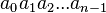$a_0a_1a_2...a_{n-1}$where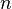$n$ is the period of the siteswap, is valid when the cardinality of the set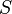$S$ (written in Set-builder notation) is equal to the period$n$ where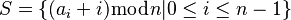$S=\{(a_i+i)\bmod n | 0\leq i\leq n-1\}$To find if a pattern is valid, first create a new sequence formed by adding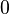$0$ to the first number,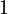$1$ to the second number,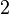$2$ to the third number and so on. Second, calculate the modulus (remainder) of each number with the period. If none of the numbers are duplicated in this final sequence, then the pattern is valid.

For example, the pattern 531 would produce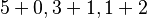$5+0,3+1,1+2$ or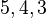$5,4,3$. Since the pattern 531 has a period of 3, The results from the previous example would produce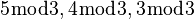$5\bmod 3,4\bmod 3,3\bmod 3$or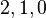$2,1,0$. In this case, 531 is valid since the numbers$2,1,0$ are all unique. Another example, 513 is an invalid pattern because the first step produces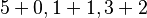$5+0,1+1,3+2$ or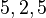$5,2,5$, the second step produces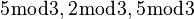$5\bmod 3,2\bmod 3,5\bmod 3$ or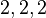$2,2,2$, and the final sequence contains at least a duplicate of one number, in this case a 2.

A synchronous siteswap is valid if

1. it only contains even numbers and
2. it can be converted into a valid vanilla siteswap using the slide property.

otherwise it is invalid.

### Swap property

New valid vanilla sequences can be generated by swapping adjacent elements from another valid vanilla siteswap sequence, adding 1 to the number being swapped to the right and subtracting 1 from the number being swapped to the left. The swap property will convert the valid sequence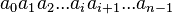${\textstyle a_0a_1a_2...a_ia_{i+1}...a_{n-1}}$ with arbitrary value$i$, to generate the new valid sequence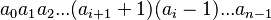$a_0a_1a_2...(a_{i+1}+1)(a_i-1)...a_{n-1}$.

For example, the swap property performed on the inner two throws of the sequence 4413 would move the 4 to the right subtracting 1 from it to become 3 and move the 1 to the left adding 1 to it to become 2. This produces the new valid siteswap pattern 4233.

### Slide property

A valid synchronous sequence can be converted to a valid asynchronous sequence and vice versa using the slide property. Given the synchronous sequence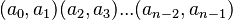$(a_0,a_1)(a_2,a_3)...(a_{n-2},a_{n-1})$ the new vanilla sequences can be formed: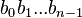${\textstyle b_0 b_1 ... b_{n-1}}$ where$b_i = \begin{cases} a_i+1, & \text{if }i\text{ is even and }a_i\text{ crosses hands} \\ a_i-1, & \text{if }i\text{ is odd and }a_i\text{ crosses hands} \\ a_i, & \text{otherwise} \end{cases}$and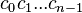$c_0 c_1 ... c_{n-1}$ where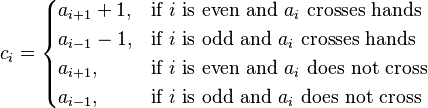$c_i = \begin{cases} a_{i+1}+1, & \text{if }i\text{ is even and }a_i\text{ crosses hands} \\ a_{i-1}-1, & \text{if }i\text{ is odd and }a_i\text{ crosses hands} \\ a_{i+1}, & \text{if }i\text{ is even and } a_i \text{ does not cross} \\ a_{i-1}, & \text{if }i\text{ is odd and } a_i \text{ does not cross}\end{cases}$The slide property gets its name by sliding the throw times of one of the hands by one time unit so that the throws align asynchronously.

For example, the siteswap (8x,4x)(4,4) would create two asynchronous (vanilla) siteswaps using the slide property: 9344 and 5744.

### Prime patterns

Siteswaps may be considered either prime or composite. A siteswap is prime if the path created in its state diagram does not traverse any state more than once. Siteswaps that are not prime are called composite.

A non-rigorous but simpler method of determining if a siteswap is prime is to try to split it into any valid shorter pattern which uses the same number of props. For example, 44404413 can be split into 4440, 441, and 3; therefore, 44404413 is composite. Another example, 441, which uses three props, is prime, as 1, 4, 41, and 44 are not valid three prop patterns (as 1/3≠3, 4/3≠3, (4+1)/3≠3, and (4+4)/3≠3). Sometimes this process does not work; for example, 153 (better known by its rotation 531) looks like it can be split into 15 and 3, but checking that the cycle has no repeating nodes in the graph traversal indicates that it is prime by the more rigorous definition.

It has been shown empirically that the longest prime siteswaps bounded by height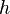$h$ contain mostly the throws$0$ and$h$. The longest prime patterns with height 22 (with 3 ball maximum), for 9 balls (with 13 maximum height), and for heights and ball counts in between, were enumerated by Jack Boyce in February 1999 using a program called jdeep. The full list of longest prime siteswaps generated by jdeep (with '0' throws represented by a '-' and maximum height throws represented by a '+') can be found here.

## Mathematical connections

### Connections to abstract algebra

Vanilla siteswap patterns may be viewed as certain elements of the affine Weyl group of type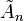${\tilde{A}}_n$. One presentation of this group is as the set of bijective functions f on the integers such that, for a fixed n: f(i + n) = f(i) + n for all integers i. If the element f satisfies the further condition that f(i) ≥ i for all i, then f corresponds to the (infinitely repeated) siteswap pattern whose ith number is f(i) − i: that is, the ball thrown at time i will land at time f(i).

### Connections to topology

A subset of these siteswap patterns naturally label strata in the positroid stratification of the Grassmannian.

## List of symbols

• Number: Relative height of a toss. 1, 2, 3...
• Brackets []: Multiplex. 33.
• Chevrons and vertical bar <|>: Simultaneous and passing patterns.
• P: Pass. <333P|333P>
• Fraction: Pass 1/y beats later. <4.5 3 3 | 3 4 3.5>
• Parentheses (): Synchronous pattern.
• *: Synchronous pattern that switches sides. (4,2x)(2x,4) = (4,2x)**x: Toss to the other hand during a synchronous pattern.

## Programs

There are many free computer programs available which simulate juggling patterns.

There are also some games to play with siteswap:Siteswap Facts for Kids. Kiddle Encyclopedia.